• 2021-04-18 06:53:34

目录

1.安装编译器

2.待续...

1.安装编译器

有多种选择，matlab自带LCC，推荐使用VC++6.0

键入：

mex-setup %有空格

mbuild -setup %有空格

若出现如下提示，建议重新安装:

错误使用 mex/mbuild，未找到支持的编译器。您可以安装免费提供的 MinGW-w64 C/C++编译器；请参阅安装 MinGW-w64 编译器。有关更多选项，请访问下载minGW-w64

安装 MinGW-w64 编译器方法等待5秒后开始下载，下载完成后

1.双击运行 MinGW-w64-install.exe /或者右键点击，选择以管理员身份运行

可以根据所要搭建的开发环境，选择不同的选项，从而下载所需的组件

Version 指MinGW-w64版本，若无特殊的需求，选择最高的版本号即可

Architecture 指系统架构是 32位 还是 64位，32位选择 i686，64位选择x86_64

Threads 指操作系统接口协议，若想要开发 Windows 程序，选择 win32 ，而 Linux,Unix,Mac OS 等其他系统程序，选择 posix

Exception 指异常处理模型(64位)

-若之前选择了64位，则可以用esh和sjlj两种异常处理模型，seh 性能比较好，但不支持 32位，sjlj 稳定性好，支持32位

-若之前选择了32位 ，则可以用dwarf和sjlj两种异常处理模型，dwarf 的性能要优于 sjlj ，但不支持 64位

Build revision 指修订版本3.点击Browse选择安装路径，完成后点击Next4.等待安装待续...

更多相关内容
• 利用MATLAB中的importdata函数读取Excel表时，运行源代码没有问题。将程序封装成exe后，该函数读取数据有问题
• 我们在程序设计中，往往会采用C#来做页面，方法用MATLAB来编写，再封装成dll供C#来调用。 但我们发现，如果电脑上没有安装MATLAB，程序则不能运行。 为解决这个问题，我们可以通过MATLAB Runtime组件来完成。 第...

我们在程序设计中，往往会采用C#来做页面，方法用MATLAB来编写，再封装成dll供C#来调用。

但我们发现，如果电脑上没有安装MATLAB，程序则不能运行。

为解决这个问题，我们可以通过MATLAB Runtime组件来完成。

第一步：登录WathWorks官网

MathWorks官网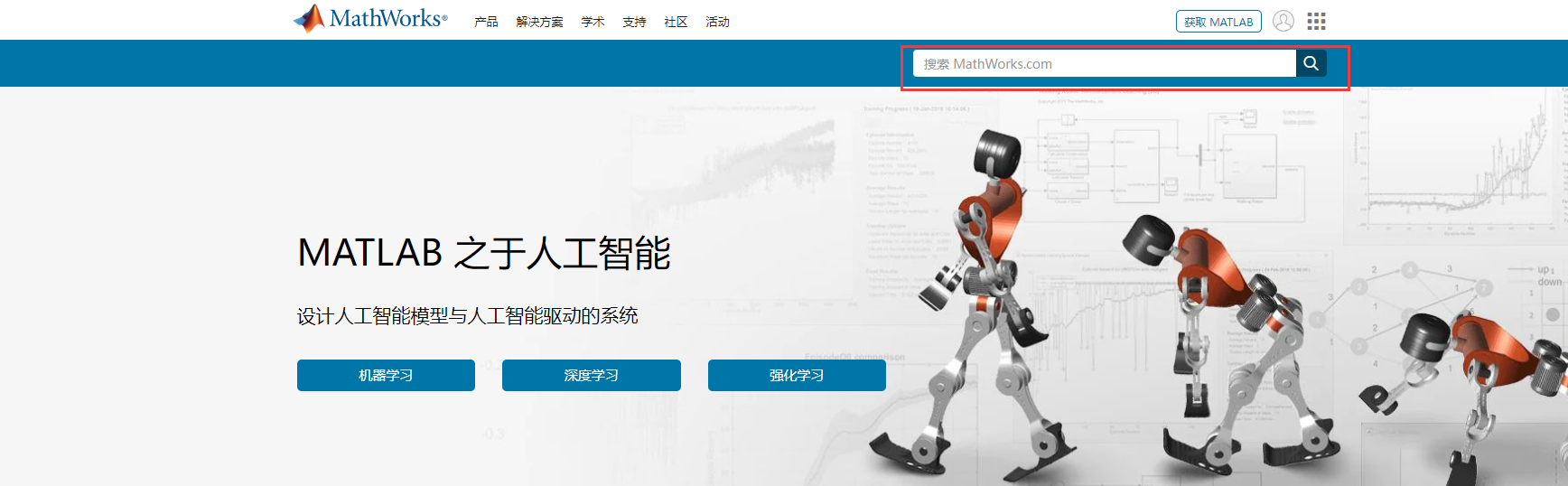第二步：搜索“Runtime”，选择第一个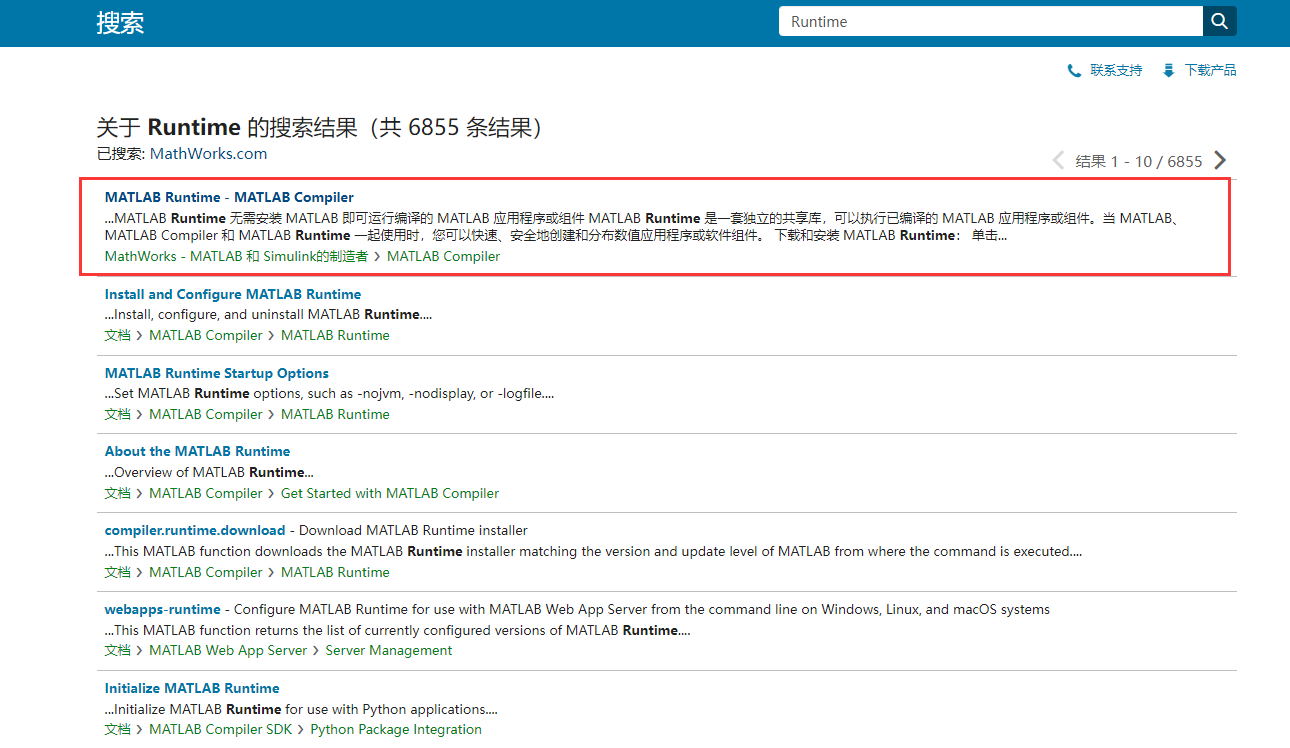第三步：选择自己对应的版本，下载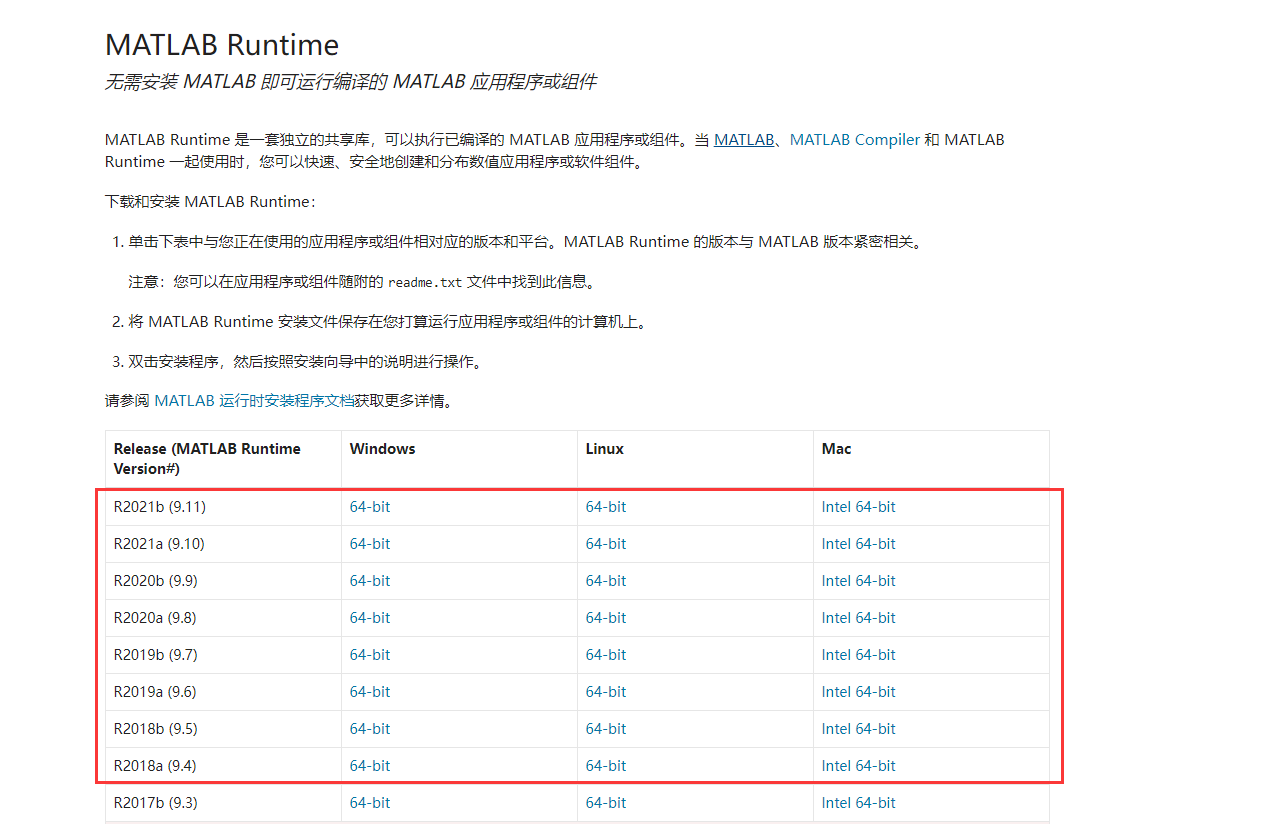第四步：解压压缩包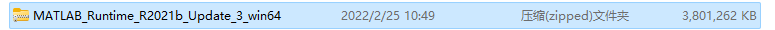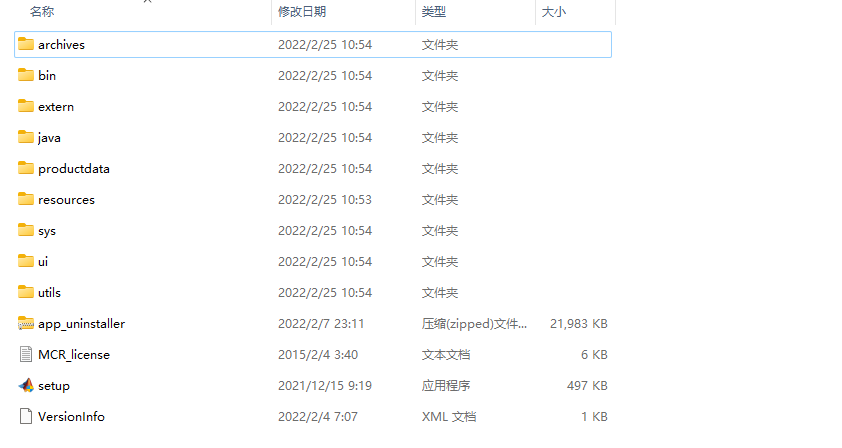第五步：双击“setup”，点击“下一步”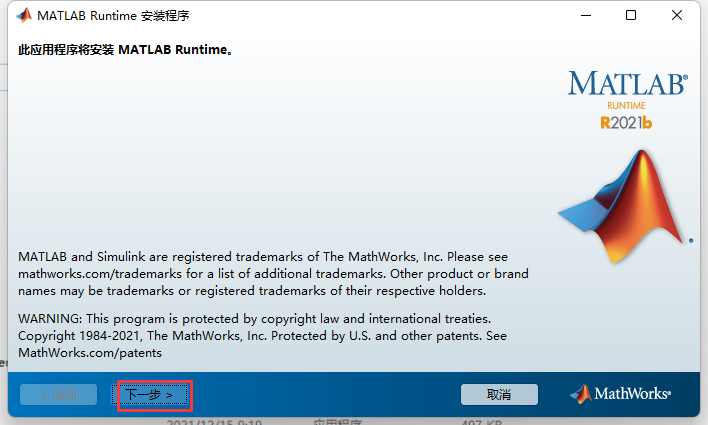接受许可协议，点击“下一步”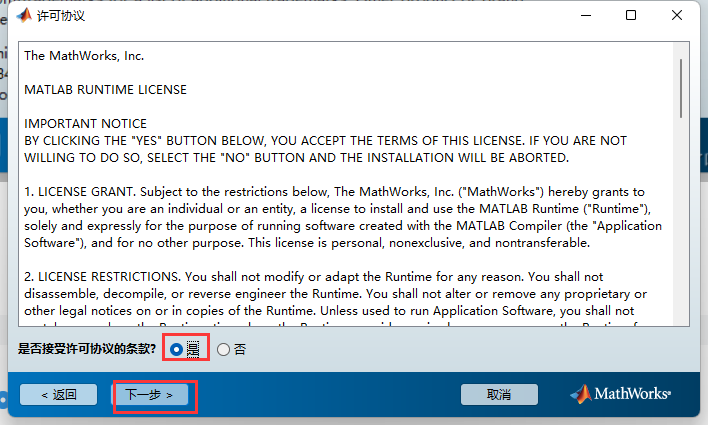点击“安装”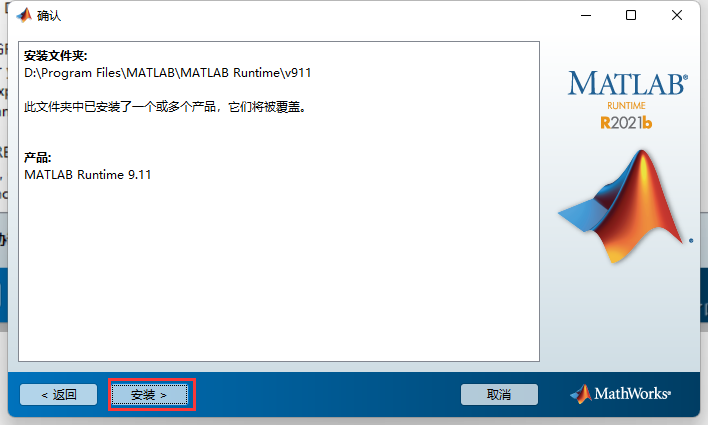选择好相应安装路径后，“安装即可”

安装好之后，程序就可以脱离MATLAB执行了

展开全文c#
• 本文主要说明了采用C#来调用MATLAB封装的dll。 本文采用的软件是MATLAB2021b(64bit) + Visual Studio 2022(.Net Framework4.8)。 第一步： 1. 创建一个C#的winform程序。 2. 修改目标平台为x64（很重要！！！...

在日常科研中中，我们往往会使用MATLAB来进行数据处理，但MATLAB的呈现就不太理想。而在工业界面设计中，我们往往会使用C#来设计页面。本文主要说明了采用C#来调用MATLAB封装的dll。

本文采用的软件是MATLAB2021b(64bit) + Visual Studio 2022(.Net Framework4.8)。

第一步：

1. 创建一个C#的winform程序。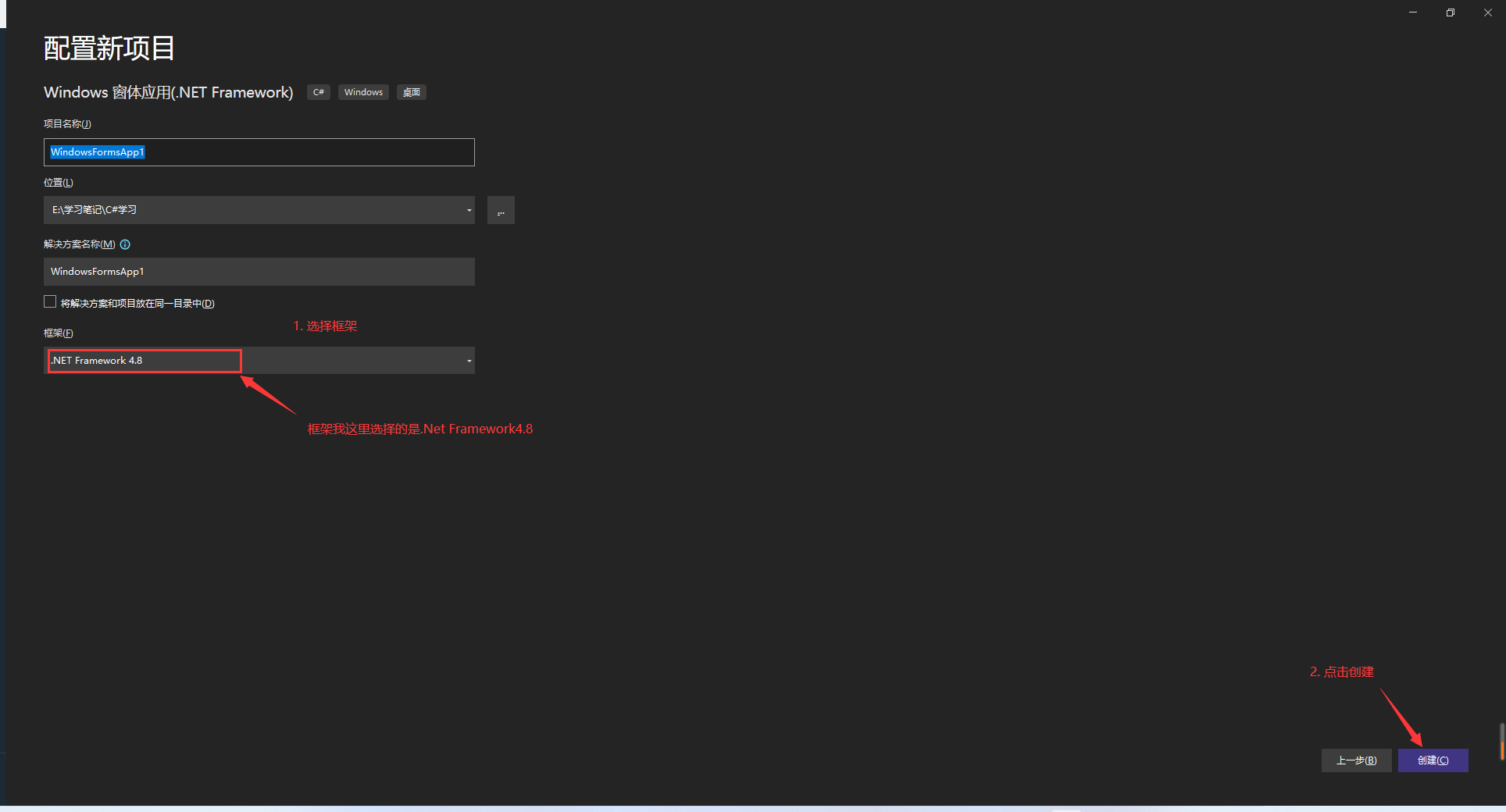2. 修改目标平台为x64（很重要！！！不改就会报错

右键项目文件—选择“属性”—选择“生成”—目标平台选择“x64”。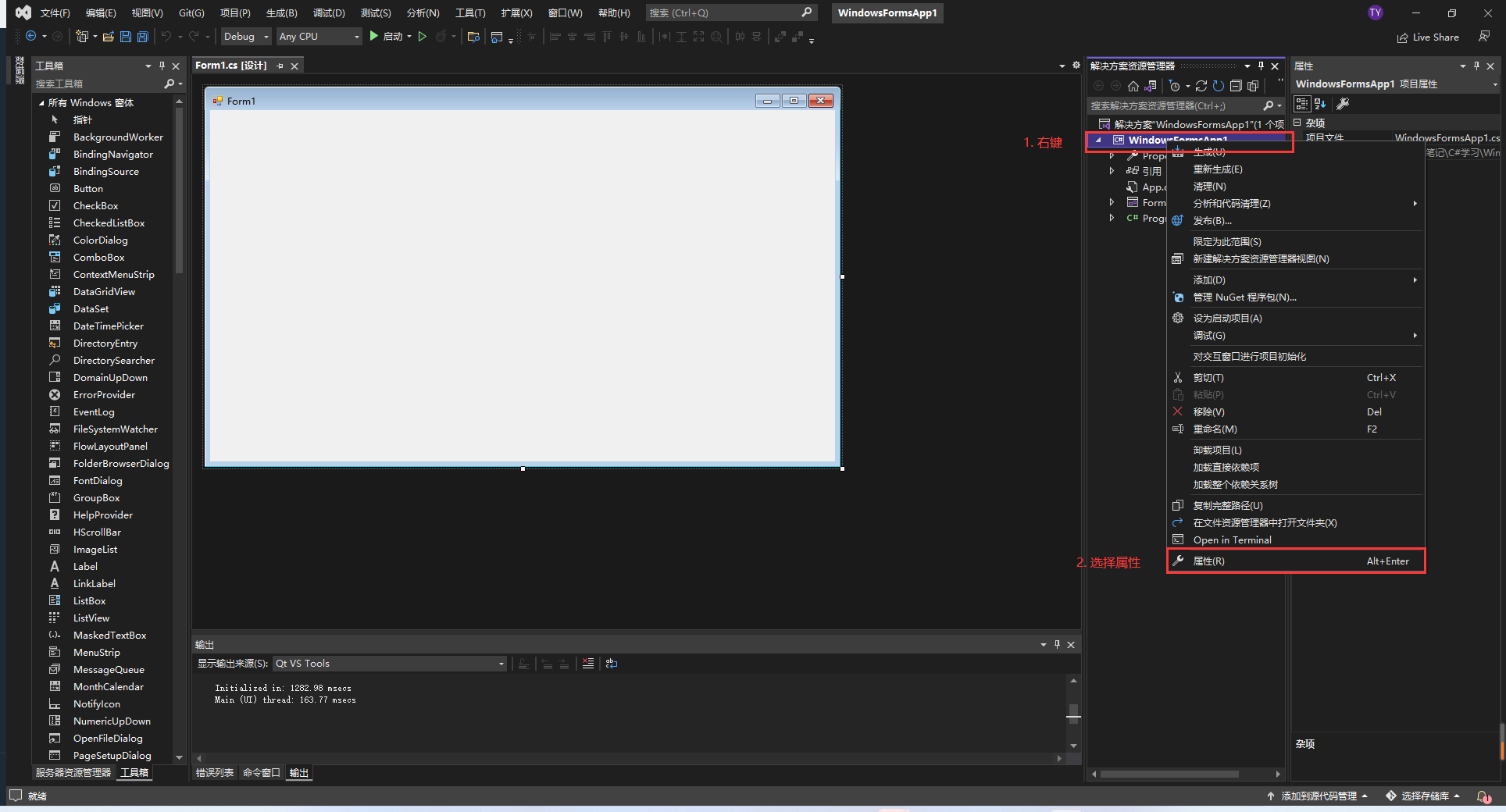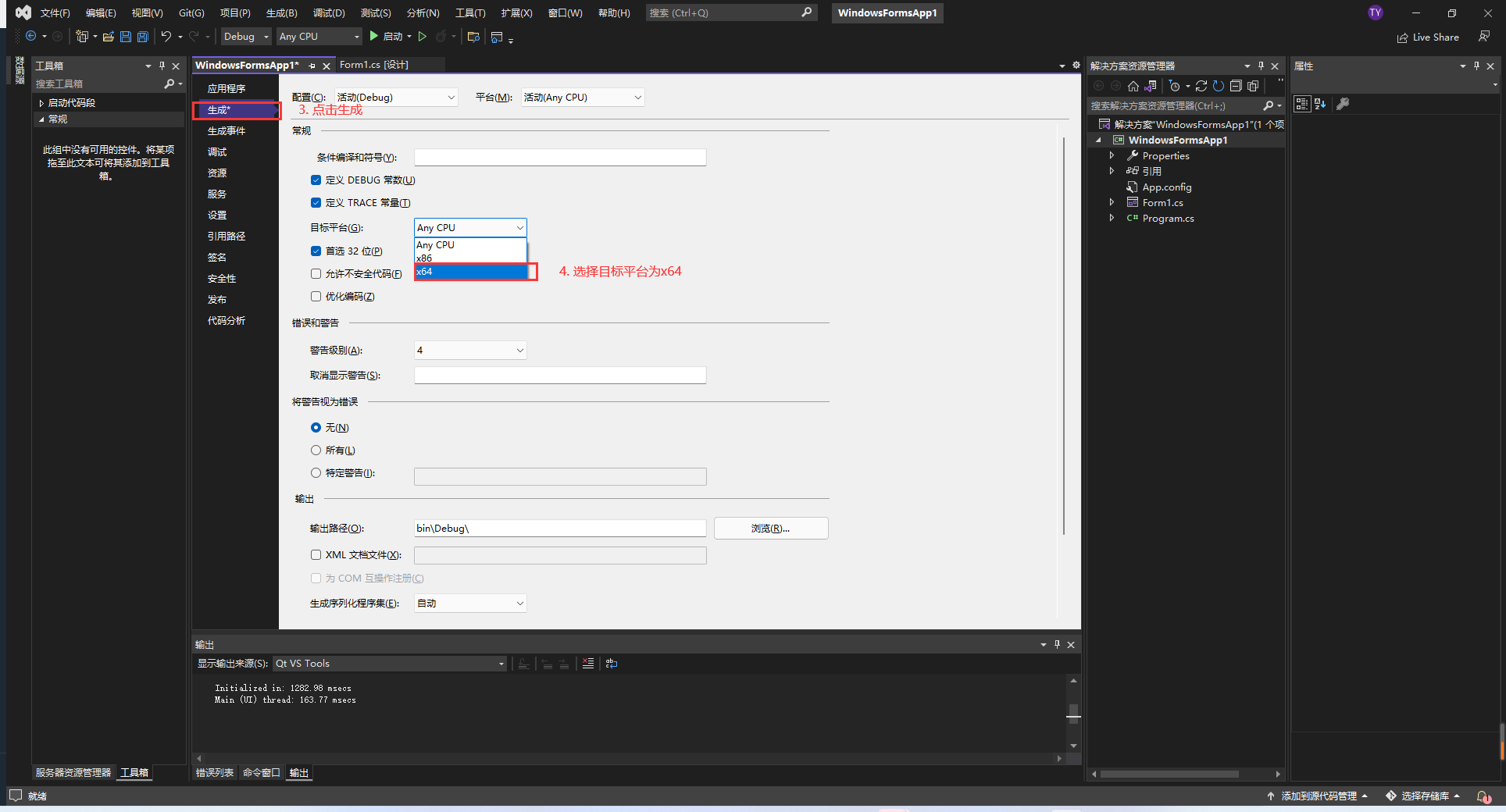3. 拖动一个button按钮和一些textBox文本框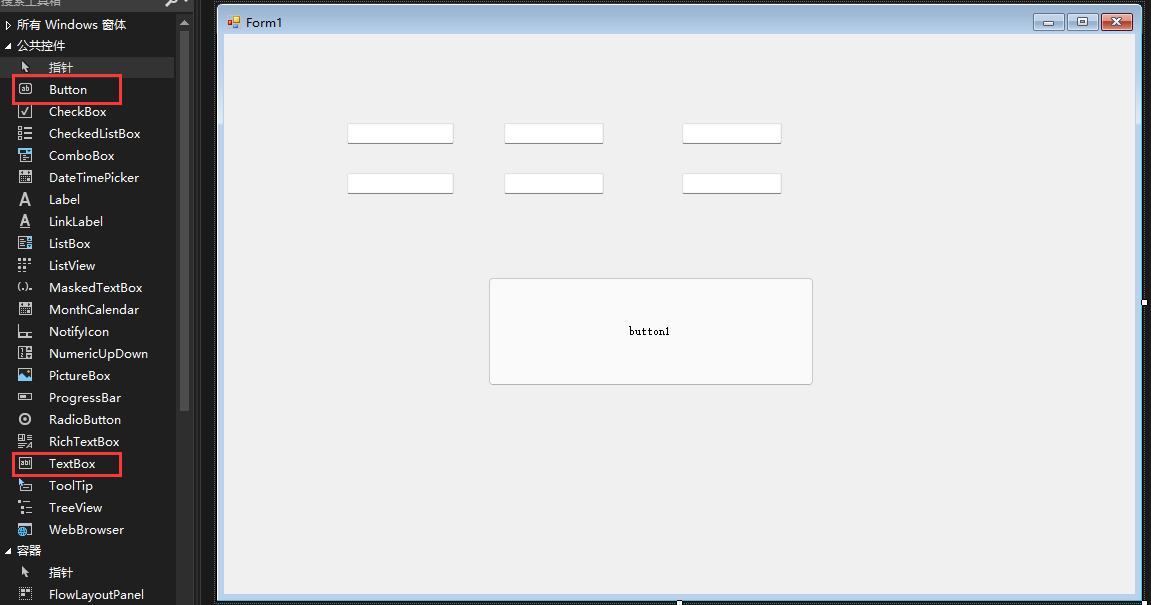4. 编写要实现的功能

在很多文章中，都喜欢用一个数据来进行数据传输，但往往在项目中都是数组数据。本文主要实现比较简单的功能，输入数据为两个C#2*3的数据，MATLAB中执行对应位置相加的结果，即输出大小也是2*3。

第二步：

1. 编写MATLAB函数，文件名为MySum.m（建议文件名与函数名一致

%% 计算两个数组按对应位置相加的结果
function out  = MySum(x1, x2)
out = x1 + x2; % x1,x2都是2*3的数组
end

2. 封装为dll文件

点击“APP”—在应用程序部署里面选择“Library Compiler”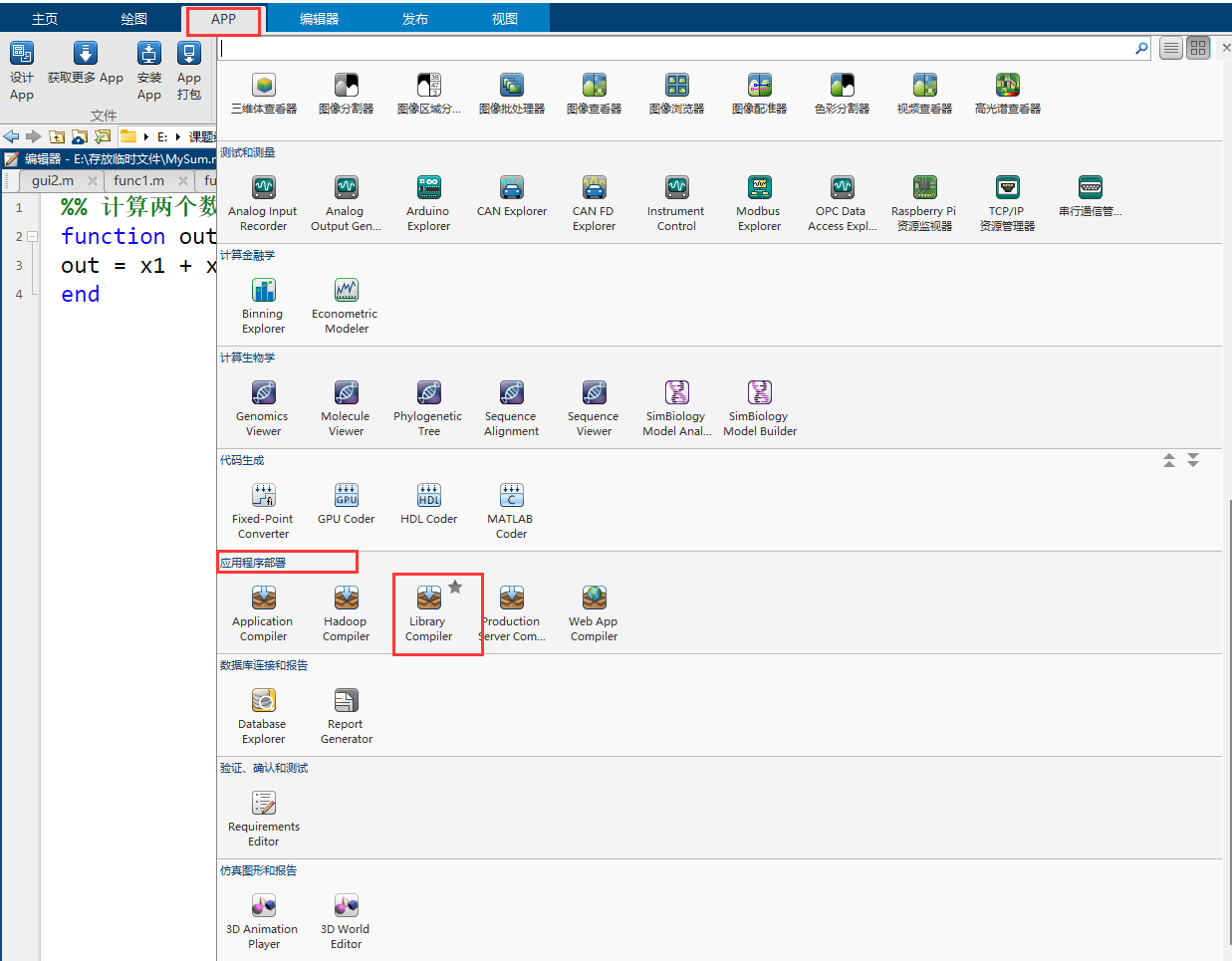在TYPE里面选择.NET Assembly、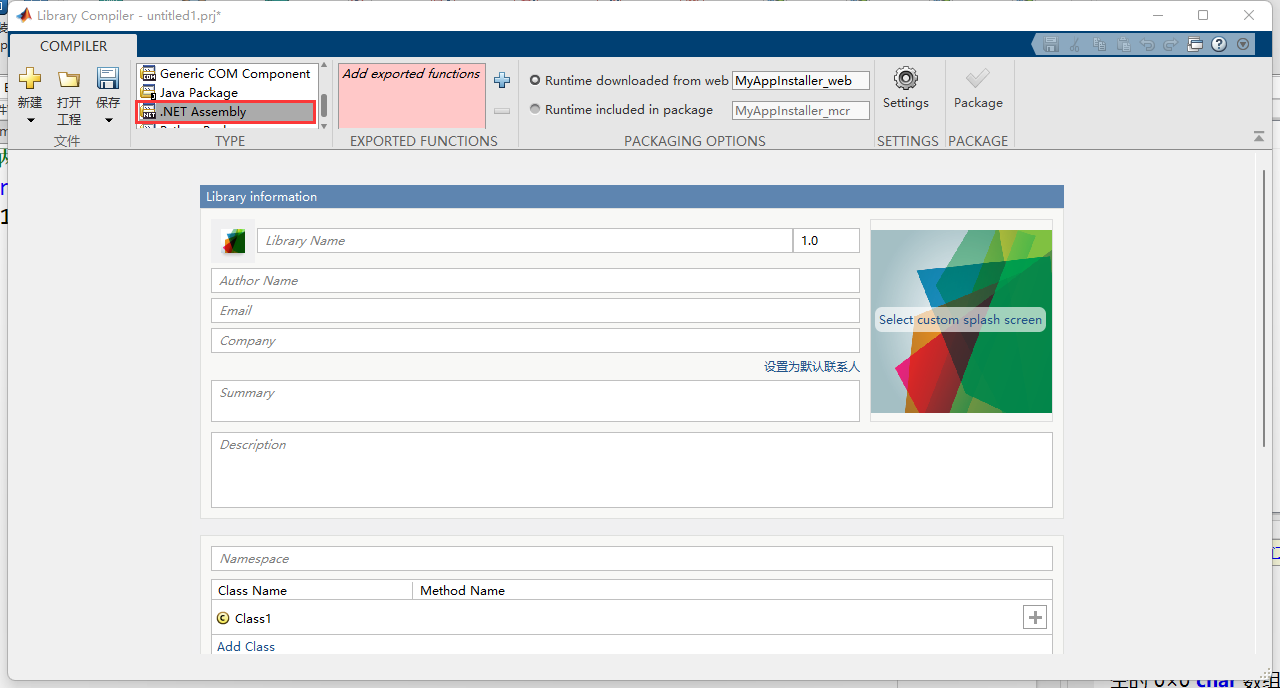点击“+”，选择我们保存的MySum.m文件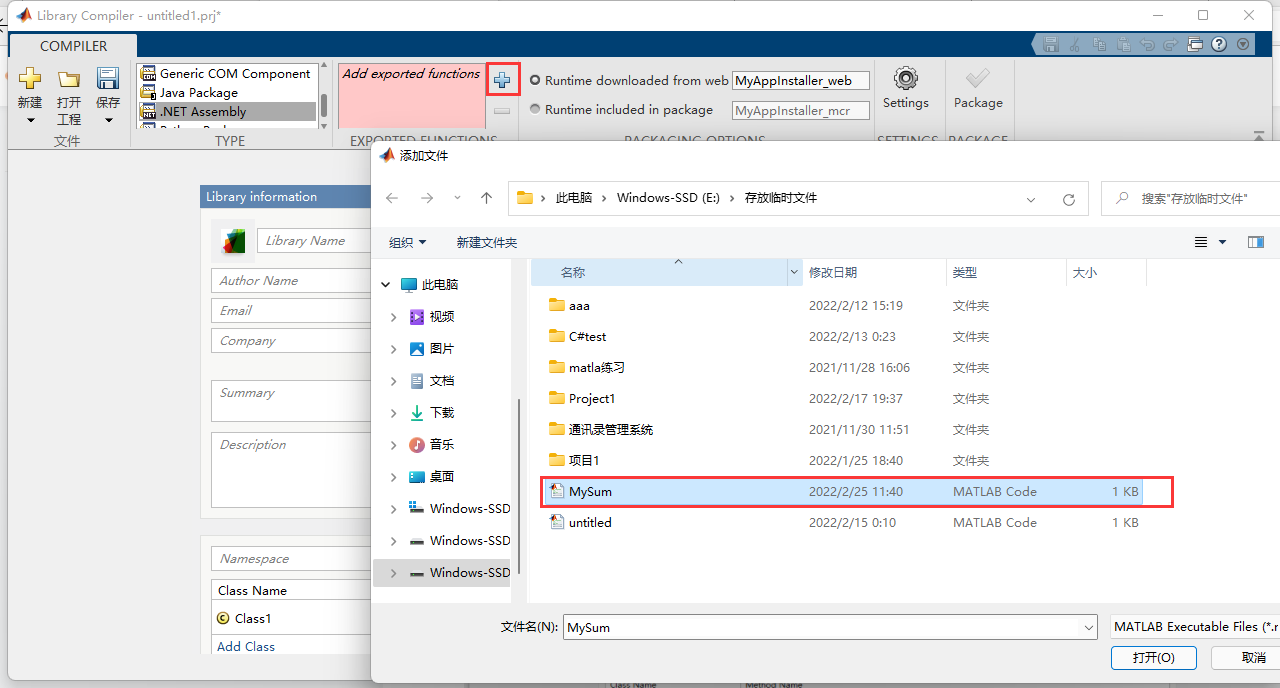点击“Package”—选择工程文件的存放路径—点击“保存”，会出现下图所示，等待打包结束。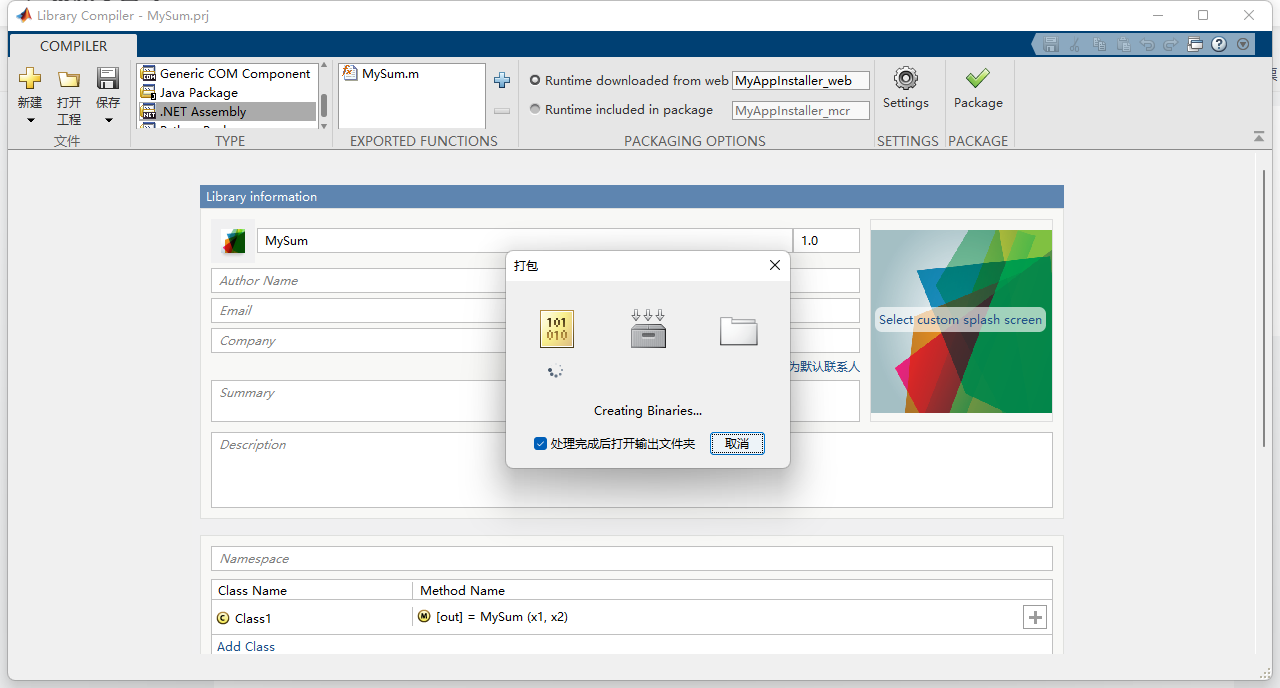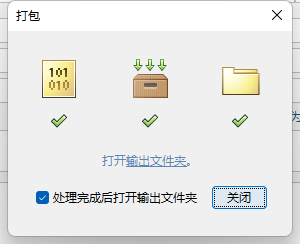成功后，选择尾缀为only的文件夹，复制里面的MySum.dll文件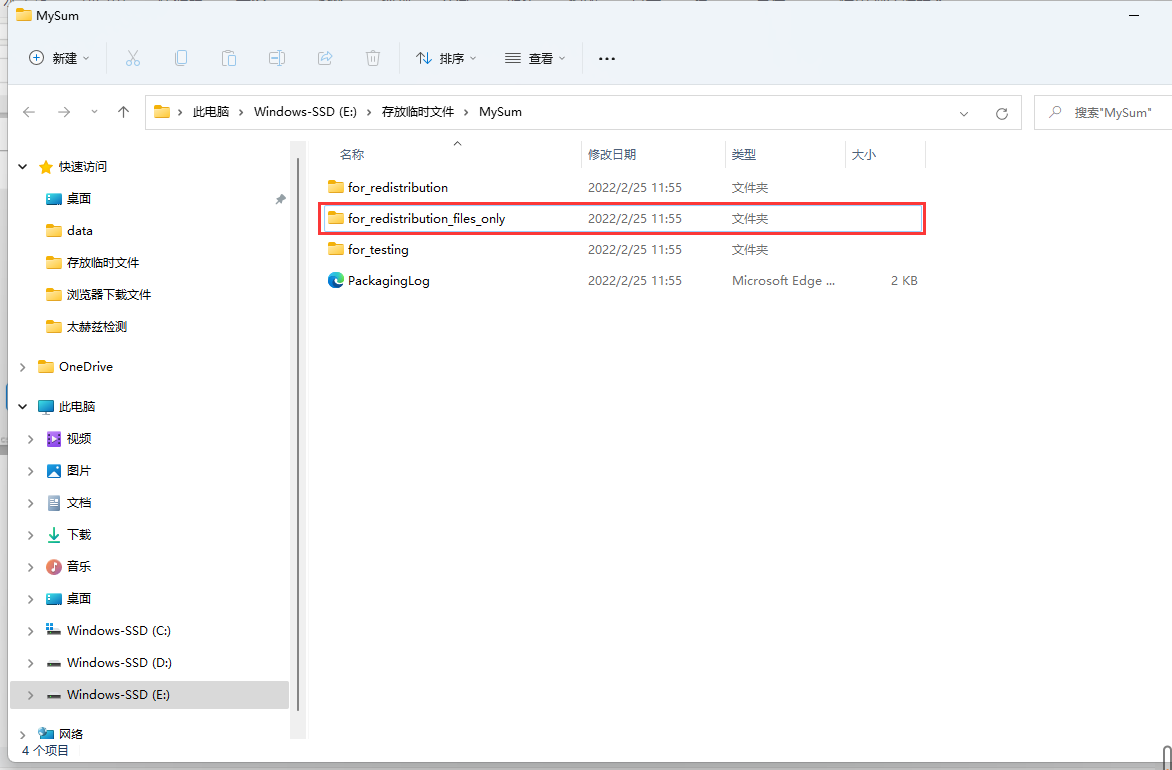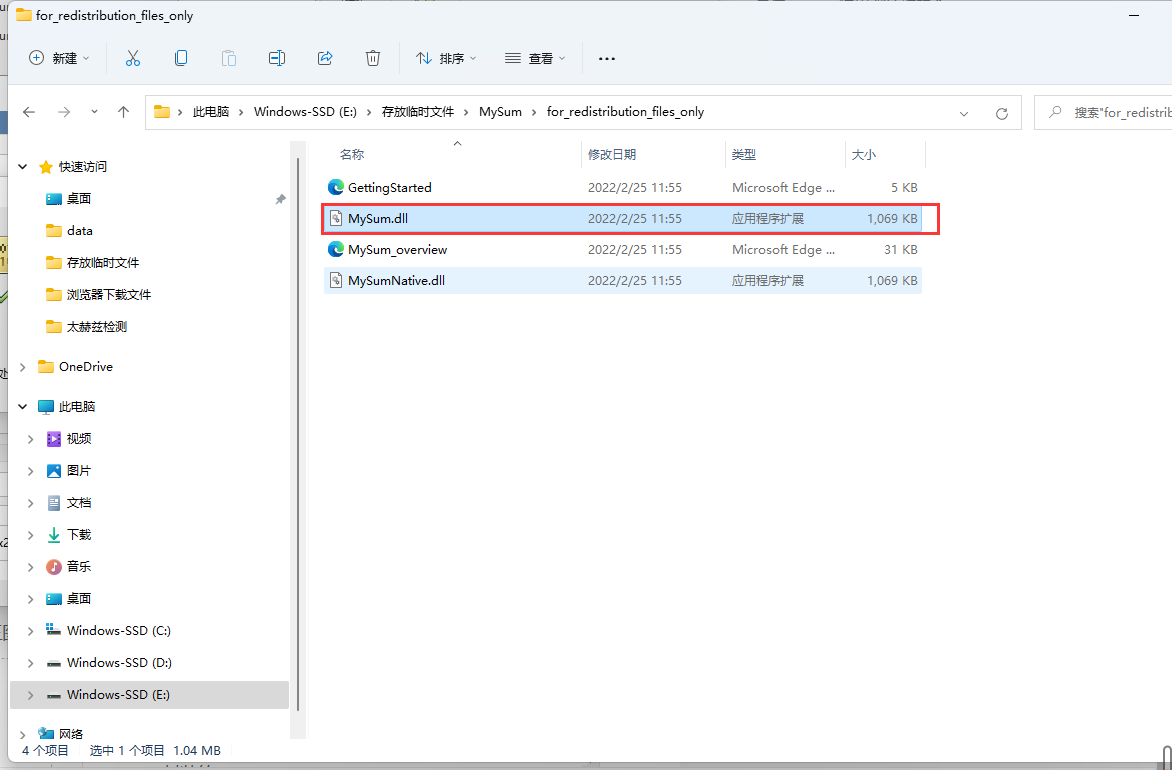将MySum.dll文件复制到C#文件目录bin\Debug文件夹下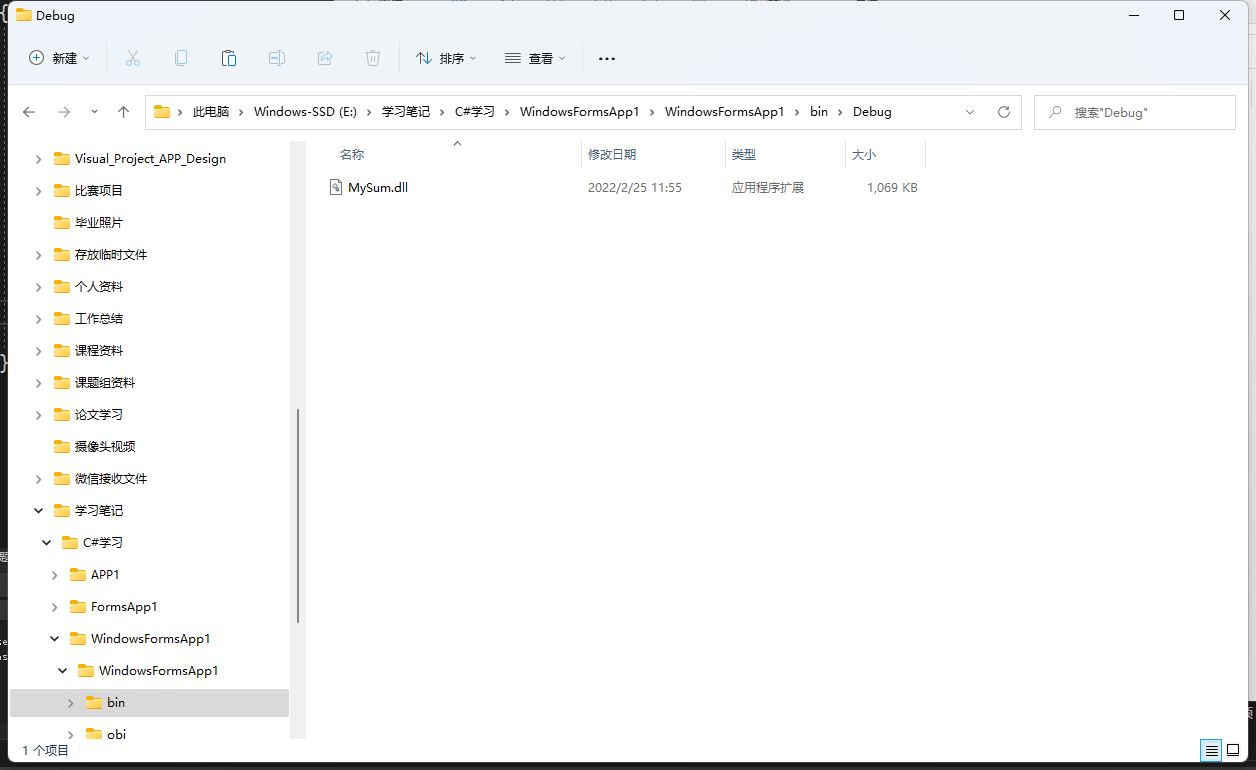将MWArray.dll文件也复制到C#文件目录bin\Debug文件夹下，MWArray.dll文件通常在MATLAB安装目录toolbox\dotnetbuilder\bin\win64\v4.0，像我的文件路径就是D:\MATLAB2021b\toolbox\dotnetbuilder\bin\win64\v4.0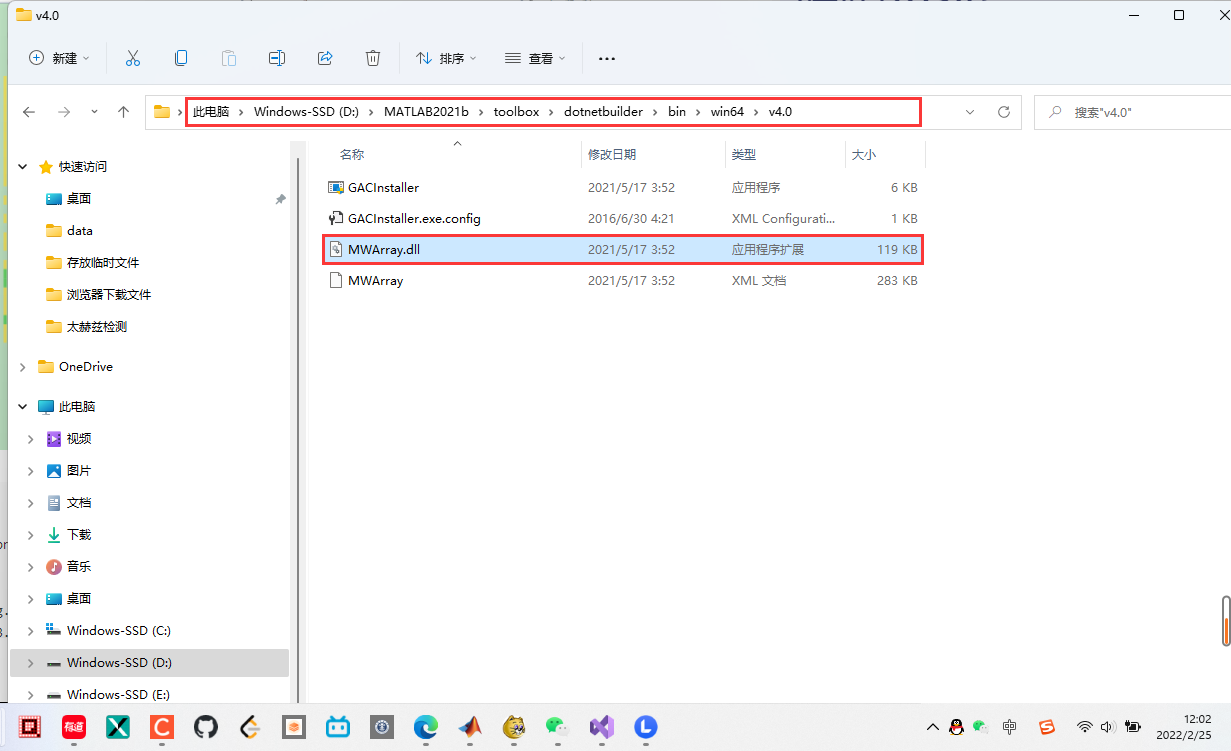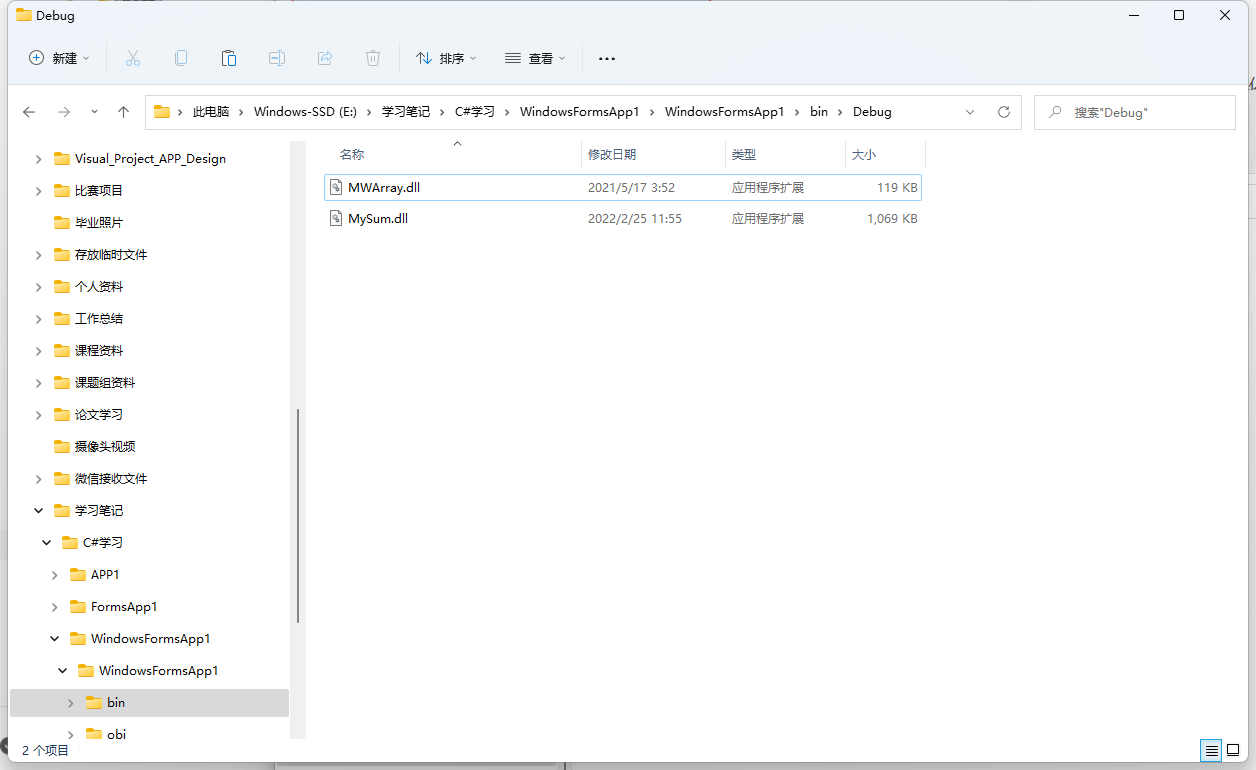第三步：编写C#程序

1. 添加引用，将MySum.dll和MWArray.dll添加到项目管理中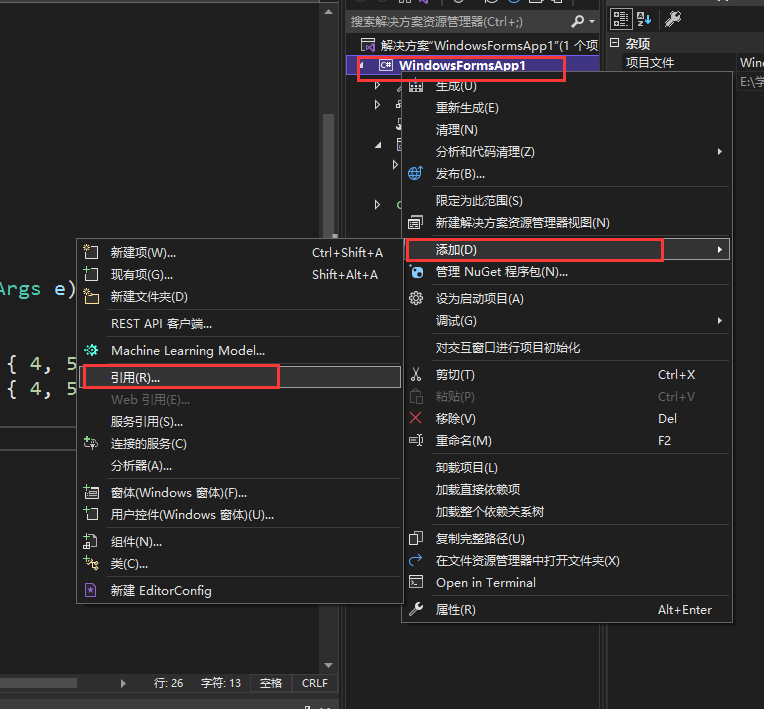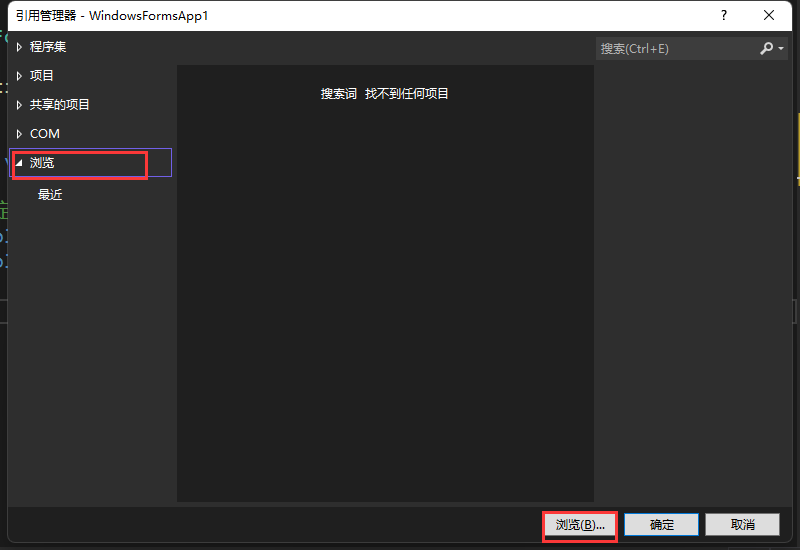选择bin\Debug文件夹下复制的两个文件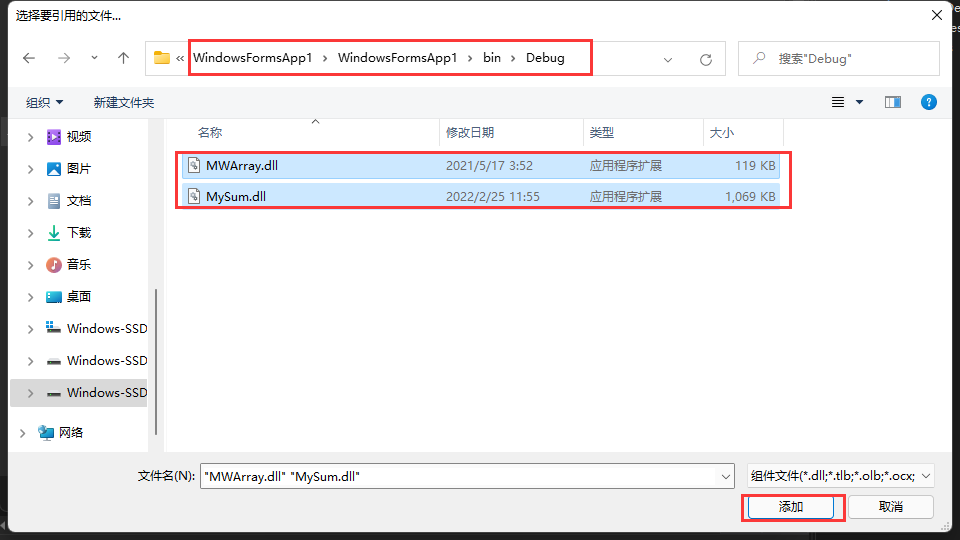添加成功后，我们能看到引用里面就已经包含了这两个文件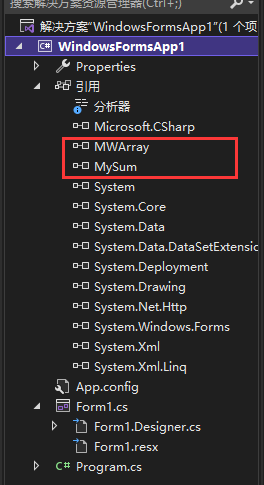2. 添加using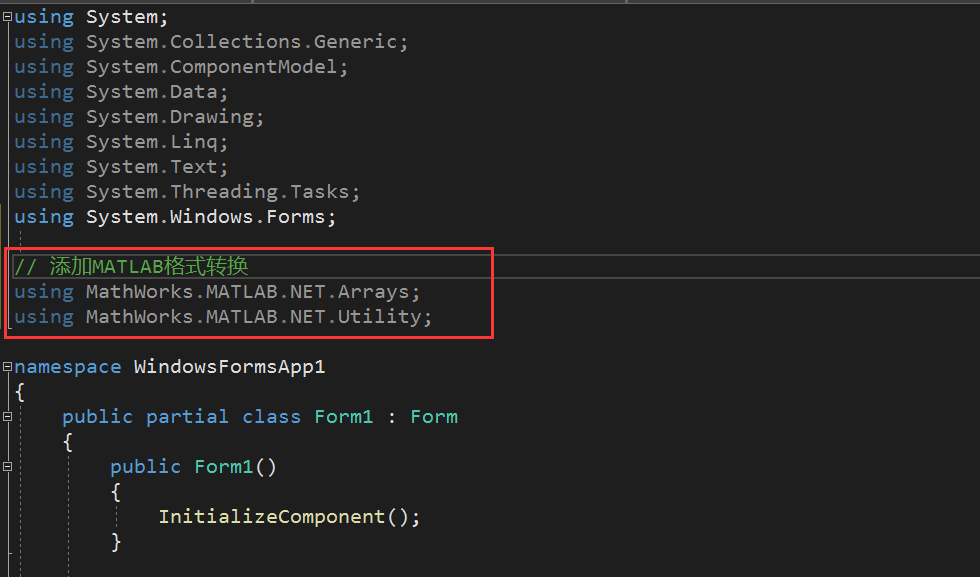3. 给一个button点击指令

        private void button1_Click(object sender, EventArgs e)
{
// 定义两个2*3的二维数组
double[,] x1 = new double[,] { { 1.25, 2.13, 3 }, { 4, 5.5764, 6 } };
double[,] x2 = new double[,] { { 1.25, 2, 3 }, { 4, 5, 6 } };

// 将C#的数据格式转换为MATLAB能够识别的数据格式
MWNumericArray arr1 = x1;
MWNumericArray arr2 = x2;

// 实例化对象
MySum.Class1 mySum = new MySum.Class1();

// 调用dll，并接收返回结果(out是关键字，不要用out来接收结果)
MWArray rst = mySum.MySum(arr1, arr2);

// 将MATLAB的数据转化为中间过渡格式
MWNumericArray result = (MWNumericArray)rst;

// 将中间过渡格式转化为C#的数据格式
double[,] csArray = (double[,])result.ToArray(MWArrayComponent.Real); // 接收数据

// 将结果展示出来（并保留两位小数）
textBox1.Text = csArray[0, 0].ToString("f2");
textBox2.Text = csArray[0, 1].ToString("f2");
textBox3.Text = csArray[0, 2].ToString("f2");
textBox4.Text = csArray[1, 0].ToString("f2");
textBox5.Text = csArray[1, 1].ToString("f2");
textBox6.Text = csArray[1, 2].ToString("f2");

}


4. 结果展示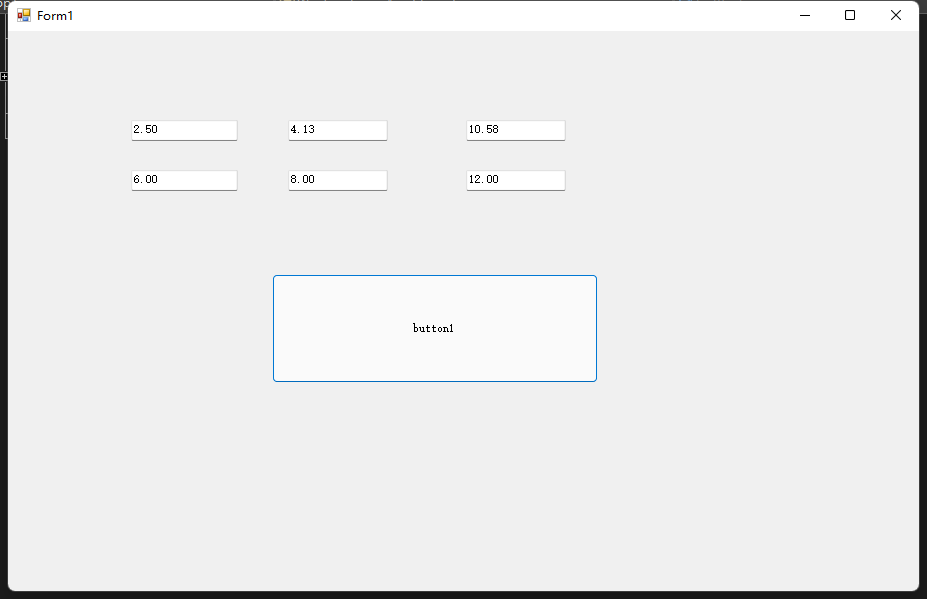C#完整代码

using System;
using System.Collections.Generic;
using System.ComponentModel;
using System.Data;
using System.Drawing;
using System.Linq;
using System.Text;
using System.Windows.Forms;

// 添加MATLAB格式转换
using MathWorks.MATLAB.NET.Arrays;
using MathWorks.MATLAB.NET.Utility;

namespace WindowsFormsApp1
{
public partial class Form1 : Form
{
public Form1()
{
InitializeComponent();
}

private void button1_Click(object sender, EventArgs e)
{
// 定义两个2*3的二维数组
double[,] x1 = new double[,] { { 1.25, 2.13, 3 }, { 4, 5.5764, 6 } };
double[,] x2 = new double[,] { { 1.25, 2, 3 }, { 4, 5, 6 } };

// 将C#的数据格式转换为MATLAB能够识别的数据格式
MWNumericArray arr1 = x1;
MWNumericArray arr2 = x2;

// 实例化对象
MySum.Class1 mySum = new MySum.Class1();

// 调用dll，并接收返回结果(out是关键字，不要用out来接收结果)
MWArray rst = mySum.MySum(arr1, arr2);

// 将MATLAB的数据转化为中间过渡格式
MWNumericArray result = (MWNumericArray)rst;

// 将中间过渡格式转化为C#的数据格式
double[,] csArray = (double[,])result.ToArray(MWArrayComponent.Real); // 接收数据

// 将结果展示出来（并保留两位小数）
textBox1.Text = csArray[0, 0].ToString("f2");
textBox2.Text = csArray[0, 1].ToString("f2");
textBox3.Text = csArray[0, 2].ToString("f2");
textBox4.Text = csArray[1, 0].ToString("f2");
textBox5.Text = csArray[1, 1].ToString("f2");
textBox6.Text = csArray[1, 2].ToString("f2");

}
}
}


展开全文c# 开发语言
• 如何把matlab代码封装用Python编程 非编程人员使用炎症数据的Python简介。 关于本课 本课教新手程序员编写模块化代码，以便使用Python进行数据分析。 但是，重点是讲授与语言无关的编程原理，例如带循环的自动化和带...
• 为什么封装好的EXE运行时报错，文件位置明明在那，请求指点怎么办！
• 这个压缩文件包含了34种现在流行的数据集降维方法，主要用于机器学习的研究。这些方法封装在了一起，可以方便地调用。
• 如何把matlab代码封装风筝 所有年龄段的编程语言。 概念 代码和数据之间没有区别。 不要重复自己。 关注点分离。 实例化。 执行顺序。 我梦想成为最好的编程语言的开发者。 样本 “评论”一些东西“单行注释”一些...
• Matlab封装BP神经网络成DLL文件并用C#调用 很抱歉，由于去年写的一篇MATLAB封装神经成DLL可能描述的不够详细，给大家带来困扰。也有人私信我让我详细说说。这几天正好有时间，我就回忆着具体写一下步骤。不太会用这...

# Matlab封装BP神经网络成DLL文件并用C#调用

很抱歉，由于去年写的一篇MATLAB封装神经成DLL可能描述的不够详细，给大家带来困扰。也有人私信我让我详细说说。这几天正好有时间，我就回忆着具体写一下步骤。不太会用这编译器，有错的地方见谅。

## 1. MATLAB训练BP神经网络

由于我当时做的数据找不到了。所以就简单的用在0到1之间随机产生数据，训练三个数之和。大致的训练步骤应该是下面的代码。

clc;
clear all;
close all;
%训练BP模型为 X+Y+Z
A = rand(1,100);
B = rand(1,100);
C = rand(1,100);
for i=1:size(A,2)
X(1,i)=A(1,i);
X(2,i)=B(1,i);
X(3,i)=C(1,i);
Y(1,i)= A(1,i) + B(1,i) + C(1,i);
end
net=newff(X,Y,,{'tansig','purelin'},'trainlm');%建立神经网络
net.trainParam.epochs=100  ;%训练次数设置
net.trainParam.goal=1e-7;%训练精度
net.trainParam.lr=0.001;%学习率设置,
net.trainParam.max_fail=100;
[net,tr]=train(net,X,Y);
save('NET_EX','net');%保存模型训练完后会产生上图的4 NET_EX.mat文件。这里面包含着训练好的神经网络。
剩下的就是验证神经网络模型。首先写个函数用来调用网络，如下：

function out=bp_out(a)
net_1 = net.net;%%提取模型内的网络
out = simcopy(net_1,a);%% 调用模型
end


代码中的simcopy函数其实就是MATLAB中的sim函数。由于要进行下一部的封装所以我把
MATLAB中自带的sim函数复制到和调用函数一个文件夹下改名字为simcopy，即图一中的3，MATLAB中sim函数在文件夹R2019a\toolbox\nnet\nnet@network中。
调用函数编写完后就是测试网络了。下图2为编写的测试函数和输出结果。

a = [0.1 ;0.3 ;0.4];
b=bp_out(a)总体来说BP的训练结束。如果我写的不清楚或者有问题可以去网上找其他人写的BP训练方法，网上很多。大致都是这个步骤，下面就是对神经网络进行DLL封装了。

## 2. MATLAB封装神经网络成DLL

MATLAB封装DLL网络上有很多具体方法，我就大致写一下。在MATLAB中输入deploytool
会出现下图3所示点击进入封装界面，如下图4。其中必须注意的是要将调用函数bp_out.m和simcopy.m一起进行封装否则调用时会报错也就是图中黄线画的地方，剩下的就是命名和DLL产生在哪个文件夹中（一般默认在MATLAB所在的当前文件夹）但图4中的标号4要记住函数名，方便在C# 中调用。到这里MATLAB封装BP神经网络DLL已经完成了。下面就是用C#调用DLL验证是否将神经网络封装成功。下面的DLL验证网络上也有很多，更详细。我这就大致写一下。

## 3. C#调用DLL验证

我用的VS编译的C#。找到MATLAB封装的DLL文件我的是bp_out.dll，和找到MWArray.dll这两个DLL文件，其中MWArray.dll我的在R2019a\toolbox\dotnetbuilder\bin\win64\v4.0中。将这两个DLL文件添加入引用中。如下图5在类的开头加上段代码

using MathWorks.MATLAB.NET.Arrays;
using MathWorks.MATLAB.NET.Utility;
using bp_out;


剩下的就是编译C#程序了，好久不碰这东西了，有点忘记C#怎么编写了，在网上找了点资料，自己写了一段程序如下段代码，可能有点繁琐。凑合着看吧。我把参考的网站贴出来。
C#调用由matlab生成的dll
C#调用Matlab生成的dll方法

using System;
using System.Collections.Generic;
using System.Linq;
using System.Text;

using MathWorks.MATLAB.NET.Arrays;
using MathWorks.MATLAB.NET.Utility;
using bp_out;
namespace test_bp
{
class Program
{
static void Main(string[] args)
{
bp_out.bp_Class1 BP_OUT = new bp_Class1();//实例化
double[,] a = new double[3, 1];
a[0, 0] = 0.1;
a[1, 0] = 0.3;
a[2, 0] = 0.4;
MWNumericArray A = new MWNumericArray(a);
MWArray out_1 = BP_OUT.bp_out(A);//调用
string Output_2 = out_1.ToString();//输出数据转化
Console.WriteLine("三个数之和 ");
Console.WriteLine(Output_2);
}
}
}



这里需要注意的如下图6所示我是64位系统。输出结果为下图7。这个C#调用MATLAB封装的DLL文件就写完了，希望对你有帮助。谢谢

展开全文c# 神经网络
• D:\R2011a\bin和E:\Microsoft Visual Studio 10.0\VC\bin，这个分别是我的机器上matlab和VS安装目录下的路径。 系统变量设置完毕后需要重新启动计算机方能生效。 二，设置matlab的编译环境 在matlab中输入命令mex...
• matlab数据平滑代码用于图割的Matlab包装器 Matlab的包装是由Shai Bagon（）编写的。 威兹曼科学研究所计算机科学与应用数学系 The core cpp application was written by Olga Veksler (available from ...
• matlab封装子系统 对于比较复杂的系统，模块化思想是很有必要的，使得思路较为清晰、错误容易排查。对复杂的系统进行仿真，如果将其中独立的功能进行封装，会显得特别清爽。这学期做了电机仿真和伺服系统仿真，特别...
• ## MATLAB封装发布exe

万次阅读 多人点赞 2016-12-06 11:42:15
从2016a开始到现在的2017，从MATLAB中创建出一个独立的应用程序有多种方法，可以用MATLAB提供的现有函数或者现有App。 本文介绍的是利用MATLAB的Application Compiler工具创建一个独立的应用程序,以下这个例子显示...
• matlab如何封装模块，子系统，MATLAB二次开发，ＧＵＩ变成，仿真
• 最近做了个项目要用到matlab做些算法处理,然后用.net项目调用这个类,我把这个matlab封装dll总结了下如下： matlab是商业数学软件，优势是在算法开发上面有很强的功能,提供了很多数学算法巩使用者调用比一般编程...
• 如何把matlab代码封装可操作性跟踪 MATLAB代码显示了可操作性跟踪任务的简单示例，在该示例中，机器人需要以其主要任务或次要目标（其中利用了机器人的零空间）来跟踪所需的可操作性椭球。 这种方法提供了转移姿势...
• 版本： linux系统的2016B版本的matlab（可以使用更高版本的matlab） 第一步：编写一个简单的matlab程序 第二步：在command window输入：deploytool 选择LIbrary Compiler ,如下图所示： 第三步：进入Matlab ...C++ linux
• 如何把matlab代码封装 新生炎症 向全球研究人员教授基础编码和数据科学技能。 该GitHub存储库生成了Software Carpentry课程网站“使用炎症数据的非程序员R简介”。 这 。 在此GitHub存储库中进行更改，使我们可以...
• 设置编译器：在确定安装好Matlab Compiler后，还需要对Compiler进行适当的配置，方法是在Matlab命令窗口输入：Mbuild –setup， 按提示选择matlab自带编译器LCC。2.将脚本编译为可执行文件：如项目文件包含：gui.m, ...
• Matlab函数封装为DLL供Cpp调用 文章目录Matlab函数封装为DLL供Cpp调用Ⅰ目标Ⅱ 学习Ⅲ 实现1. Matlab 函数封装为DLL2. vs2015中C++调用Matlab DLL步骤Ⅳ 问题解决Ⅴ 后续可能有用信息 Ⅰ目标 Matlab函数封装为DLL，...cpp
• MATLAB程序封装成EXE文件1.设置编译器：首先需要配置自己的Matlab Compiler，Matlab Compiler的作用是将程序编译成为机器可以直接执行的程序。配置Compiler 的方法是在Matlab命令窗口输入：mbuild -setup，按提示...
• 编译环境：matlab 2007b，winxp1.设置编译器：在确定安装好MatlabCompiler后，还需要对Compiler进行适当的配置，方法是在Matlab命令窗口输入：Mbuild –setup，按提示选择matlab自带编译器LCC。2.将脚本编译为可执行...
• 该资源包括一套MATLAB的教学视频，同时包含了MATLAB的软件，可以直接下载使用。视频教程来源于网络
• 本文简单介绍一下Matlab（2021b）子系统封装时比较有意思的两个选项，即check box与popup menu，封装效果如下图1所示： 图1 封装效果图 对于本例这两项封装，总结为：check box勾选即为布尔型1，未勾选为0；...
• 介绍在MATLAB中将m文件封装为jar包,以供在Java中调用。...

# matlab封装matlab 订阅